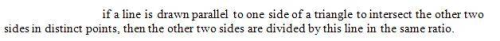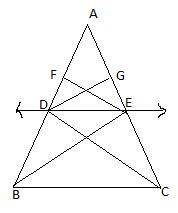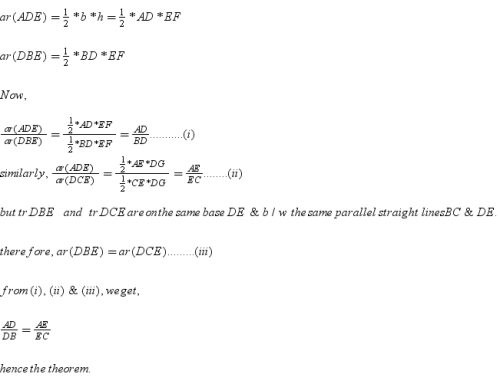Courses

Basic Proportionality Theorem Notes - Class 10

Class 10: Basic Proportionality Theorem Notes - Class 10

The document Basic Proportionality Theorem Notes - Class 10 is a part of Class 10 category.
All you need of Class 10 at this link: Class 10

Prove that [basic proportional theorem]?
Ref: https://edurev.in/question/617932/Prove-that-basic-proportional-theorem-

Basic Proportionality Theorem(Thales Theorem) -Given - In triangle ABC,  DE is parallel to BC. DE bisects AB at D and AC at E.

Construction - Join BE and CD and draw EF is perpendicular to AB and DG is perpendicular to AC.

Proof -

As,  EF is perpendicular to AB, EF is the height of tr's ADE and DBE.The document Basic Proportionality Theorem Notes - Class 10 is a part of Class 10 category.
All you need of Class 10 at this link: Class 10Use Code STAYHOME200 and get INR 200 additional OFF Use Coupon Code

Top Courses for Class 10Top Courses for Class 10Track your progress, build streaks, highlight & save important lessons and more!

,

,

,

,

,

,

,

,

,

,

,

,

,

,

,

,

,

,

,

,

,

;